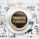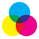# Computing FIR Filters Using Arrays [WMA Example]

1919 visualizzazioni
Over the years, many FIR filters have been proposed by the Pine community, with the standard way of computing them being `for` loops. The arrival of arrays allows for a new, more efficient way to compute them.

This script provides a template showing how you can compute FIR filters using Pine arrays.

FIR Filters

FIR stands for "Finite Impulse Response", and is associated with types of filters whose impulse response reaches a steady state.

FIR filters are calculated using convolution, or more simply put, using a weighted sum between a set of filter coefficients and past input values over a finite window.

In Pine, FIR filters are generally computed inside a `for` loop executing three processes:

1- Computing the coefficients.
2- Summing all the computed coefficients.
3- Performing the weighted sum between the inputs values and the computed coefficients.

Then we divide the result of our weighted sum by the sum of the coefficients obtained in step 2.

Because the computations inside the `for` loop execute on each bar, execution time can be significant when the calculation of coefficients is complex. This is where arrays are handy, as we can compute the coefficients just once, store them into an array, and use them in a weighted sum without the need to recalculate them over and over. This drastically reduces the computation time required to calculate a FIR filter.

The new `array.sum()` function helps eliminate step 2, thus further decreasing computation time.

How to Use This Template

All you need to do is to put the code that computes your coefficients in the first `for` loop (variable `w`). If the code that computes your coefficients contains more than one line, just make sure your final coefficient is placed in variable `w` (or change the `value` argument in `array.push()`). Another option is to declare a function that computes the coefficients and use it instead of variable `w`.
Script open-source

Nello spirito di condivisione promosso da TradingView, l'autore (al quale vanno i nostri ringraziamenti) ha deciso di pubblicare questo script in modalità open-source, così che chiunque possa comprenderlo e testarlo. Puoi utilizzarlo gratuitamente, ma il riutilizzo del codice è subordinato al rispetto del Regolamento. Per aggiungerlo al grafico, mettilo tra i preferiti.

Vuoi usare questo script sui tuoi grafici?
Tools and ideas for all Pine coders: http://www.pinecoders.com
Our Pine FAQ & Code: http://www.pinecoders.com/faq_and_code/

## CommentiJust reading the name was enough to know it was alex haha

Good job!
RispondiPratik_4Clover
@Pratik_4Clover, Yup the whole time I was reading the explanation I was thinking that this would be his jam !!! then boom he's in the script it self :) lol
It is a lot of fun to learn form and follow the leaders in this pinescript community

Thank you @PineCoders @alexgrover for publishing this awesome script
Rispondi
Profilo Impostazioni profilo Account e fatturazione Amici invitati Coin I miei ticket di supporto Centro di supporto Messaggi Privati Chat Esci